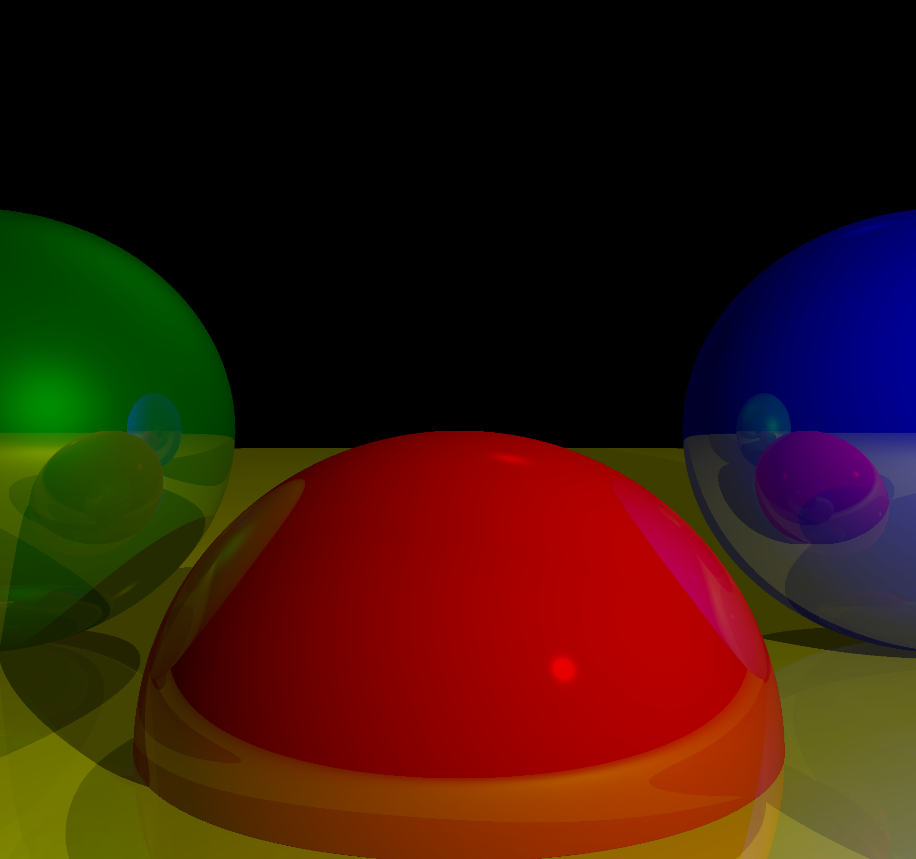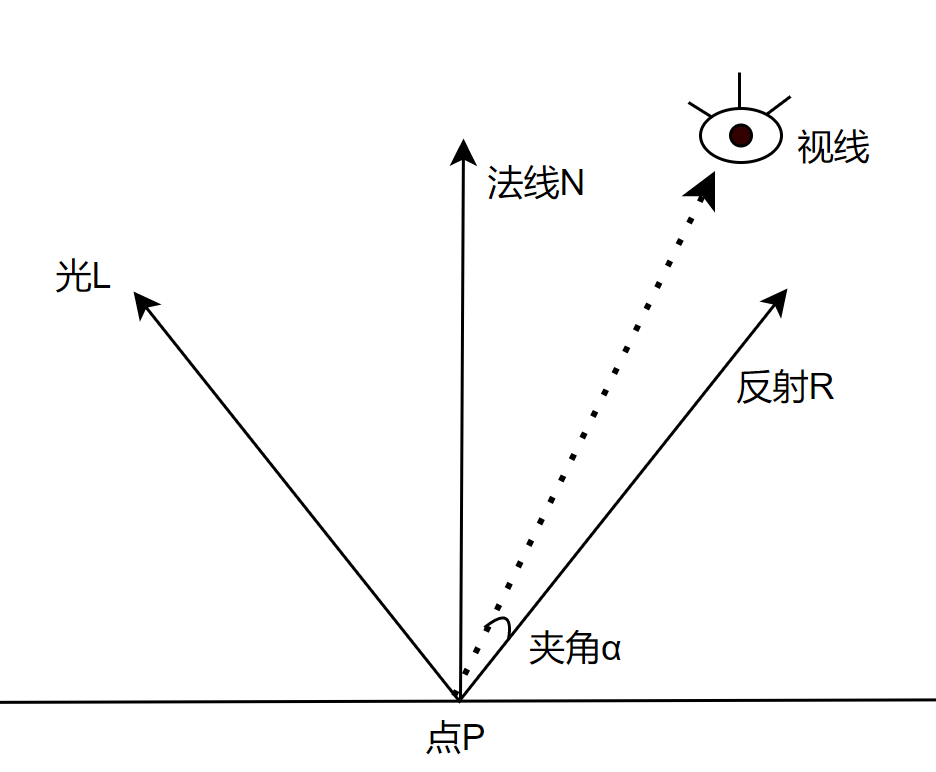# 添加镜面反射# 镜面反射## 分析什么意思呢，就是此时我发出射线看到P，然后我们应该继续发射射线追踪光L，看看是否是其他点反射过来的。

## 代码：

      Vector3D reflectiveray(Vector3D R, Vector3D N)
{
return 2 * N * Vector3D.DotProduct(N, R) - R;
}

  Color tracrray(Vector3D origin, Vector3D dline, double min, double max, int deepth = 3)
{
double closet = double.PositiveInfinity;
var clp = closestIntersection(origin, dline, min, max);

if (clp == null)
{
return Colors.Black;
}
closet = clp.Closet;
var claset_sharp = clp.Claset_sharp;
var p = origin + (closet * dline);
var n = p - claset_sharp.center;
n = n / n.Length;
var cl = ComputeLighting(p, n, -dline, claset_sharp.specular);
//保证颜色上下限正确
var M = Color.FromRgb((byte)(Math.Min(255, Math.Max(0, cl * claset_sharp.color.R))),
(byte)(Math.Min(255, Math.Max(0, (cl * claset_sharp.color.G)))),
(byte)(Math.Min(255, Math.Max(0, (cl * claset_sharp.color.B)))));
if (claset_sharp.reflective <= 0 || deepth <= 0)
{
return M;
}
var r = reflectiveray(-dline, n);
var color = tracrray(p, r, 0.001, double.PositiveInfinity, deepth - 1);
var k = 1 - claset_sharp.reflective;
var rgb1 = Math.Min(255, Math.Max(0, M.R * k));
var rgb2 = Math.Min(255, Math.Max(0, M.G * k));
var rgb3 = Math.Min(255, Math.Max(0, M.B * k));
var m2 = Color.Multiply(M, (float)k);
var m3 = Color.Multiply(color, (float)claset_sharp.reflective);
return m4;
}

void Start()
{
WriteableBitmap.Lock();
for (double x = 0 - cw / 2; x < cw / 2; x++)
{
for (double y = 0 - ch / 2; y < ch / 2; y++)
{
var D = canvastoviewport(new Point(x, y));
var color = tracrray(new Vector3D(), D, 1, double.MaxValue, 10);
var p = MidPoint(new Point(x, y));

byte[] colorData = { color.B, color.G, color.R, color.A };

int stride = (WriteableBitmap.PixelWidth * WriteableBitmap.Format.BitsPerPixel) / 8;
WriteableBitmap.WritePixels(new Int32Rect((int)p.X, (int)p.Y, 1, 1), colorData, stride, 0);
}
}
WriteableBitmap.Unlock();

}

posted @ 2022-06-28 17:49  ARM830  阅读(126)  评论(2编辑  收藏  举报